# Circles 2

Calculate the area bounded by the circumscribed and inscribed circle in triangle with sides 12 cm, 14 cm, 18 cm.

S =  209.4 cm2

### Step-by-step explanation: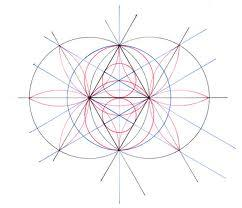We will be pleased if You send us any improvements to this math problem. Thank you!Tips to related online calculators
See also our trigonometric triangle calculator.

#### You need to know the following knowledge to solve this word math problem:

We encourage you to watch this tutorial video on this math problem:

## Related math problems and questions:

• Square and circlesSquare with sides 83 cm is circumscribed and inscribed with circles. Determine the radiuses of both circles.
• Triangle ABCCalculate the sides of triangle ABC with area 1404 cm2 and if a: b: c = 12:7:18
• Annulus from triangleCalculate the content of the area bounded by a circle circumscribed and a circle inscribed by a triangle with sides a = 25mm, b = 29mm, c = 36mm
• CirclesThe area of a circle inscribed in a square is 14. What is the area of a circle circumscribed around a square?
• Sss triangleCalculate the area and heights in the triangle ABC by sides a = 8cm, b = 11cm, c = 12cm
• Cathethus and the inscribed circleIn a right triangle is given one cathethus long 14 cm and the radius of the inscribed circle of 5 cm. Calculate the area of this right triangle.
• TriangleThe triangle has known all three sides: a=5.5 m, b=5.3 m, c= 7.8 m. Calculate area of ​this triangle.
• Desribed circle to rectangle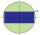Rectangle with sides 6 cm and 4 cm was circumscribed circle. What part of the content of the circle determined by the circumscribed circle occupies a rectangle? Express in perctentages(%).
• Rhombus and inscribed circleIt is given a rhombus with side a = 6 cm and the radius of the inscribed circle r = 2 cm. Calculate the length of its two diagonals.
• Inscribed circleXYZ is right triangle with right angle at the vertex X that has inscribed circle with a radius 5 cm. Determine area of the triangle XYZ if XZ = 14 cm.
• Annular areaThe square with side a = 1 is inscribed and circumscribed by circles. Find the annular area.
• Sides of the triangleCalculate triangle sides where its area is S = 84 cm2 and a = x, b = x + 1, xc = x + 2
• Ratio of sidesCalculate the area of a circle with the same circumference as the circumference of the rectangle inscribed with a circle with a radius of r 9 cm so that its sides are in ratio 2 to 7.
• Rectangle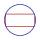The rectangle is 18 cm long and 10 cm wide. Determine the diameter of the circle circumscribed to the rectangle.
• TrapezoidCalculate the area of trapezoid ABCD with sides |AD|= 68 cm, |DC|=35 cm, |CB|=12 cm, |AB|=35 cm..
• Two circles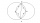Two circles with the same radius r = 1 are given. The center of the second circle lies on the circumference of the first. What is the area of a square inscribed in the intersection of given circles?
• Circle inscribed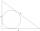Calculate the perimeter and area of a circle inscribed in a triangle measuring 3 , 4 and 5 cm.